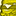# QlikView App Dev

Discussion Board for collaboration related to QlikView App Development.

Announcements
Join this live chat April 6, 10AM EST - QlikView to Qlik Sense REGISTER
cancel
Showing results for
Search instead for
Did you mean:Contributor III

## Calculation Problam

Hi all,

i calculated the  accuracy field in the next way:

round(Sum([orders]/SUM(TOTAL([orders]))*[ ACCURACY BY UNIT]))/100

my problem is that i need to represent for the calculate field (A B & C) the number that reflects  total accuracy  for each calculate type(A B  & C).

now   i  get 59% as total for A B & C toghther .

Does somone have an idea how to get totals as :  A - 59%  insted of 20% B- 59% INSTED OF 20%  C- 60% insted of 20%.

when i remove the    ACCURACY BY UNIT field i get totals as A - 20% B- 20% C- 20%

I need to show totals such as : A - 59% B-59% C - 60%

thanks

Roee

1 ReplyMVP

Try round(sum(ORDERS*[ACCURACY BY UNIT])/sum(TOTAL <CALCULATE> ORDERS))

talk is cheap, supply exceeds demand